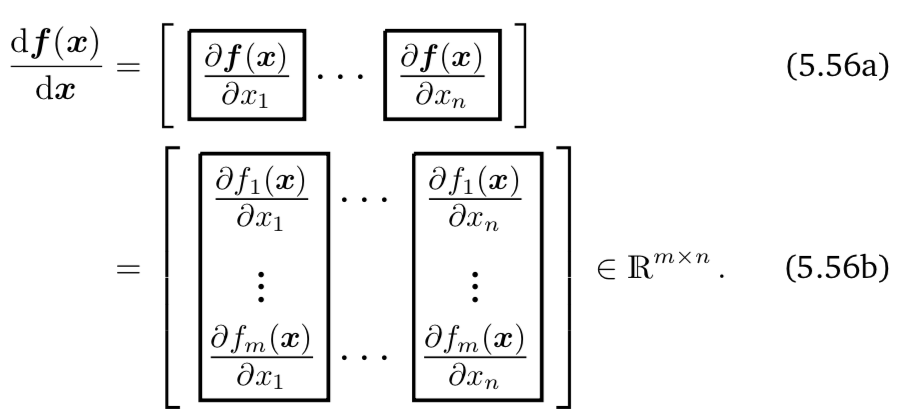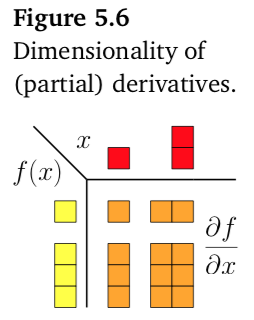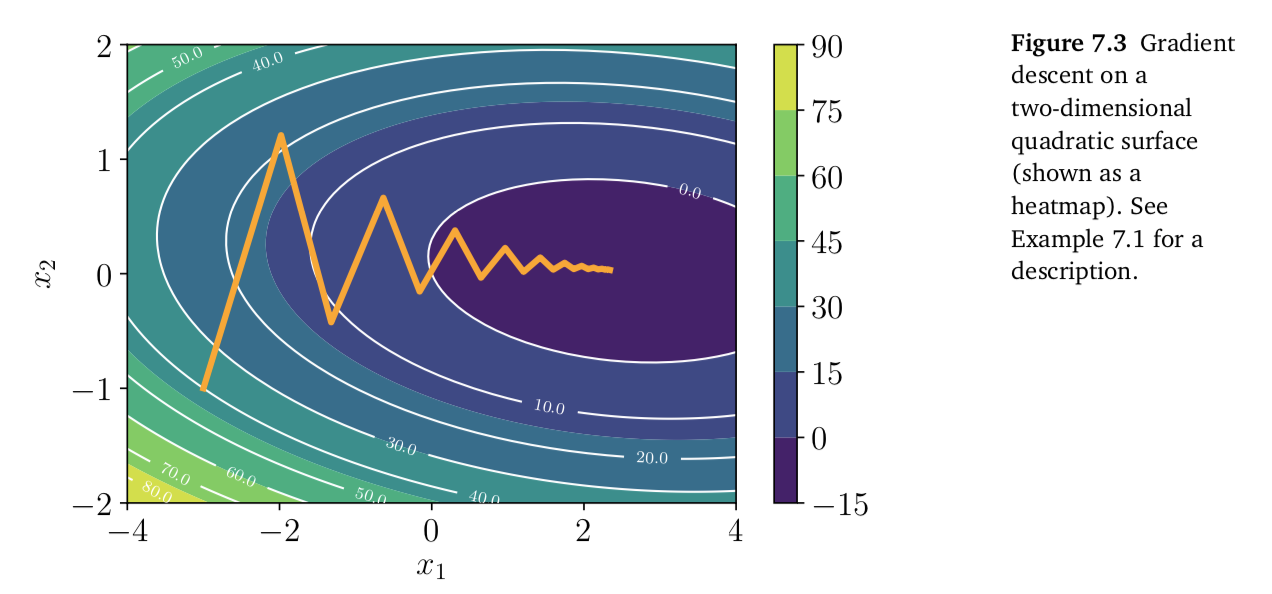# Chapter 11 Vector Calculus

• MML, chapter 5

## 11.1 理論

${\bf f(\theta)=}\begin{bmatrix}f_{1}({\bf \theta})\\ \vdots\\ f_{m}({\bf \theta}) \end{bmatrix}\in\mathbb{R}^{m\times1}$

• $$m$$個函數

${\bf f(\theta)}=\begin{bmatrix} a1*\theta_1+a2*\theta_2+a3*\theta_3\\ b1*\theta_1^2+b2*\theta_2^3 \end{bmatrix}$

def f(theta):
"""
theta: np.ndarray. represents [theta1, theta2]
return <np.ndarray>
"""

numpy有一系列的polynomial routines

$\frac{\partial{\bf f(\theta)}}{\partial \theta_{i}}{\bf =}\begin{bmatrix}\frac{\partial f_{1}({\bf \theta})}{\partial \theta_{i}}\\ \vdots\\ \frac{\partial f_{m}({\bf \theta})}{\partial \theta_{i}} \end{bmatrix}\in\mathbb{R}^{m\times1}$Figure 11.1: 向量函數維度

### 11.1.1 Jacobian

The collection of all first-order partial deriva- tives of a vector-valued function is called the Jacobian.

$\min_{\theta} f(\theta)$

• $$\nabla_{\theta}f(\theta)$$ gives that direction that can increases f value. To decrease value, we need to go $$-\nabla_{\theta}f(\theta)$$ direction。

• Only direction, but how long? $$-\gamma \nabla_{\theta}f(\theta)$$

$\theta_{i+1}=\theta_{i}-\gamma \nabla_{\theta}f(\theta_i)$

$\theta_{i+1}=\theta_{i}-\gamma_i \nabla_{\theta}f(\theta_i)$

• $$\gamma_i$$ is called the learning rate.
• When the function value increases after a gradient step, the step-size was too large. Undo the step and decrease the step-size.
• When the function value decreases the step could have been larger. Try to increase the step-size.

### 11.2.3 Gradient Descent With Momentum

As illustrated in Figure 7.3, the convergence of gradient descent may be very slow if the curvature of the optimization surface is such that there are regions that are poorly scaled.

knitr::include_graphics(
"fig/fig7_3.png"
)$\theta_{i+1}=\theta_{i}-\gamma_i \nabla_{\theta}f(\theta_i)+ \alpha \Delta \theta_i$

$f(\theta)=\sum_{n=1}^N f(\theta | \bf x_n)$

$\begin{eqnarray} \theta_{i+1} &=& \theta_{i}-\gamma \nabla_{\theta}f(\theta_i)\\ &=& \theta_{i}-\gamma \sum_{n=1}^N \nabla_{\theta}f(\theta_i|\bf x_n) \end{eqnarray}$

• N can be huge.

• Randomly draw a mini-batch $$B_i$$ from training data. And consider the following loss function instead:

$f_{B_i}(\theta) =\sum_{\bf x_n\in B_{i}}f(\theta|\bf x_n)$

$\theta_{i+1}=\theta_{i}-\gamma \sum_{\bf x_n\in B_{i}} \nabla_{\theta}f(\theta_i|\bf x_n)$

• Batch Gradient Descent. Batch Size = Size of Training Set

• Stochastic Gradient Descent. Batch Size = 1

• Mini-Batch Gradient Descent. 1 < Batch Size < Size of Training Set

We will divided training set into several mini batches. And use each through several iterations.

• Epochs